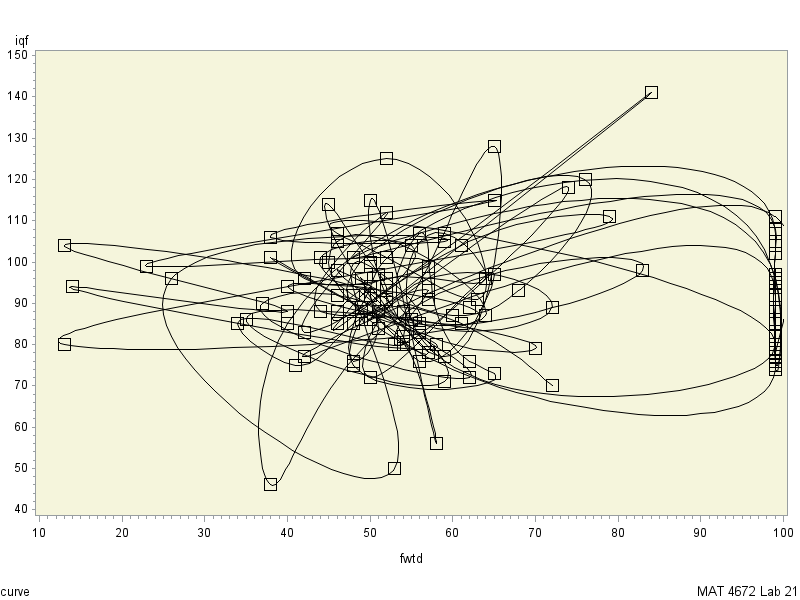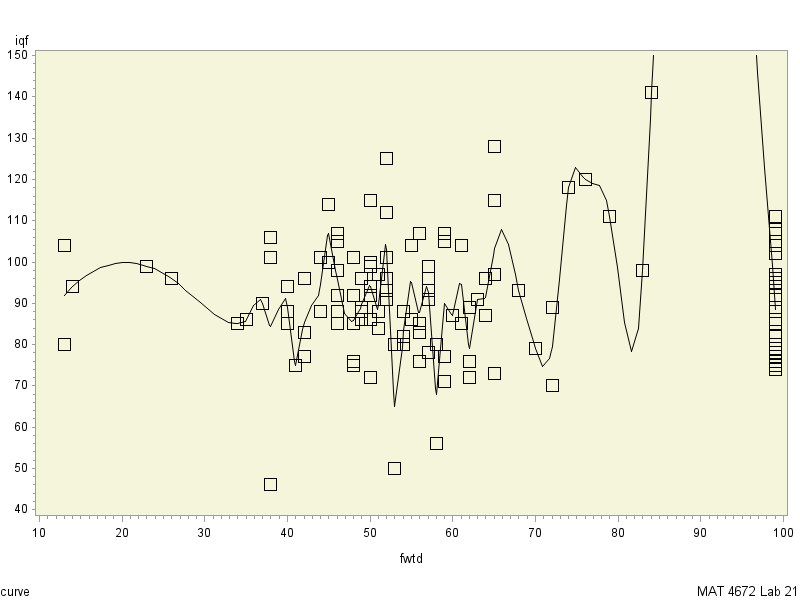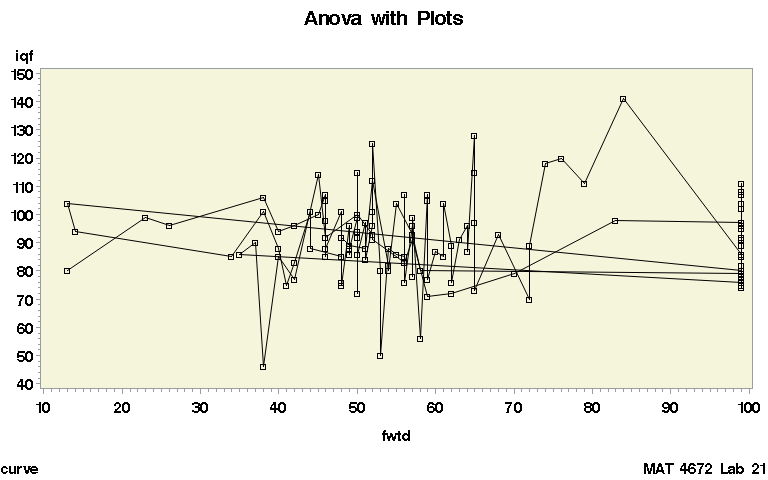## PROC gplot

Hi,

I try to plot graph using PROC GPLOT:

```PROC GPLOT DATA = lead1;

footnote j=l 'curve'
j=r 'MAT 4672 Lab 21';
plot iqf*fwtd=3/ frame cframe=beige;;
RUN;
QUIT;```

and i got thisHow make it nice and sorted.

P.S

I tried that

```PROC SORT data = lead1;
by fwtd;
run;

footnote j=l 'curve'
j=r 'MAT 4672 Lab 21';
plot iqf*fwtd=3/ frame cframe=beige;;
RUN;
QUIT;```

and got that:` `

7 REPLIES 7

## Re: PROC gplot

What are you expecting to get? And what do you think the = 3 means in your plot statement?

## Re: PROC gplot

1)3 means squares instead just dots
2) i am expecting graph through all points(squares) and sorted by X axis like
https://encrypted-tbn2.gstatic.com/images?q=tbn:ANd9GcSnEg3_Ebu_DNwEWswUg8aygvFFBKT61C9alWAMhujgcFzP...

## Re: PROC gplot

Yeah, I don't think 3 does what you think it does. I can't test it, but I think you want something like this, where you use the symbol statement to define the symbol (square) and the interpol=j to join the points.

``````
symbol1 interpol=j value='square';

footnote j=l 'curve'
j=r 'MAT 4672 Lab 21';
plot iqf*fwtd / frame cframe=beige;;
RUN;
QUIT;``````

Alternatively you can use the SGPLOT procedures which I find easier to navigate:

``````proc sgplot data=sashelp.stocks (where=(stock="IBM"));
scatter x=date y=open / markerattrs=(symbol='square' color='beige');
series x=date y=open / lineattrs=(color='beige');
run;quit;``````

## Re: PROC gplot

@bigban777 wrote:
1)3 means squares instead just dots
2) i am expecting graph through all points(squares) and sorted by X axis like
https://encrypted-tbn2.gstatic.com/images?q=tbn:ANd9GcSnEg3_Ebu_DNwEWswUg8aygvFFBKT61C9alWAMhujgcFzP...

3 means to use Symbol3 statement for the display. Yours has some sort of interpolation option (INTERPOL= or I=) other than JOIN to "fit" a curve through the data. I would guess either SM Spline or L.

If you want to connect the points you need I=Join.

## Re: PROC gplotI believe x should be sorted

See attached file

## Re: PROC gplot

Post your code/log if it doesn't work in the message, preferably in a code window.

## Re: PROC gplot

Hi,

I would suggest 1) move over to sgplot and graph template language, you will find more options/better outputs and 2) check out Sanjay's blog:

http://blogs.sas.com/content/graphicallyspeaking/

Which has many thousands of examples on all kinds of graphs, you will find what you are looking for there.

Discussion stats
• 7 replies
• 1483 views
• 1 like
• 4 in conversation